# WBBSE Class 9 Physical Science Solutions Chapter 6 Heat

Detailed explanations in West Bengal Board Class 9 Physical Science Book Solutions Chapter 6 Heat offer valuable context and analysis.

## WBBSE Class 9 Physical Science Chapter 6 Question Answer – Heat

Question 1.
What is the unit of heat in CGS system ?
Calorie is the unit of heat in CGS system.

Question 2.
What is the unit of heat in SI system ?
In SI system, the unit of heat is Joule.

Question 3.
What is steam point?
Steam point is the boiling point of water under normal pressure.Question 4.
What is 1 calorie?
1 calorie is defined as the heat required to raise the temperature of lg of water through one degree Celsius.

Question 5.
What is the unit of specific heat in CGS system ?
The unit of specific heat in CGS system is cal/g/°C

Question 6.
What is the unit of specific heat in SI system ?
The unit of specific heat in SI system is J/kg/K.

Question 7.
What is fundamental interval ?
It is the range of temperature between the upper and lower fixed points in the scale of temperature.Question 8.
What are the scales of temperature ?
There are three scales of temperature —

• Celsius scale
• Fahrenheit scale &
• Kelvin scale.

Question 9.
What is the value of 100°C in Fahrenheit scale ?
In Fahrenheit scale the temperature is 212°F.

Question 10.
What are the factors for determining the quantities of heat in a body ?
The quantity of heat depends upon the following factors :

• temperature
• mass and
• material of the body.

Question 11.
Is heat a vector quantity ?
No, heat is a scalar quantity.

Question 12.
What is the relation between calorie and joule ?
1 calorie = 4.18 joule

Question 13.
What is the principle of calorimetry ?
The principle of calorimetry is :
Heat absorbed by the cold body
= Heat given out by the hot body.Question 14.
Is there any change of temperature during the change of state ?
There is no change of temperature during the change of state.

Question 15.
Which temperature is lower — 0°C or 0°F ?
0°F is lower than 0°C.

Question 16.
What are the lower fixed point and upper fixed point of thermometer in Celsius scale ?
Lower fixed point is 0°C and upper fixed point is 100°C.

Question 17.
What are the lower fixed point and upper fixed point of thermometer in Fahrenheit scale ?
Lower fixed point is 32°F and upper fixed point is 212°F.

Question 18.
What is the fundamental interval of Celsius scale ?
The fundamental interval is divided in 100 equal parts in Celsius scale.

Question 19.
What is the fundamental interval of Fahrenheit scale ?
The fundamental interval is divided into 180 equal parts in Fahrenheit scale

Question 20.
What is the melting point of ice in Kelvin scale ?
Melting point of ice in Kelvin scale is 273K.Question 21.
What type of scale is used in clinical thermometer ?
Generally Fahrenheit scale is used in clinical thermometer.

Question 22.
Which temperature is same in both Celsius scale and Fahrenheit scale ?
– 40° temperature will read same both in Celsius scale and Fahrenheit scale.

Question 23.
Which substance has the highest specific heat ?
Water has the highest specific heat.

Question 24.
What is specific heat capacity ?
The specific heat in SI system is called specific heat capacity.

Question 25.
What is the value of specific heat capacity of pure water ?
The specific heat capacity of pure water is 4200 J/kg/K.

Question 26.
Is specific heat of solid greater than that of liquid ?
Generally specific heat of solid has lower value than that of liquid.

Question 27.
What is the normal temperature of human body ?
The normal temperature of human body is 98-4°F or 36-9°C.Question 28.
If a substance has thermal capacity 100 calorie, what will be its water equivalent ?
If the substance has thermal capacity 100 calorie, then water equivalent of the substance will be 100 g.

Question 29.
What is the relation between Celsius scale and Kelvin scale ?
If a temperature in Kelvin scale is T K and the temperature in Celsius scale is t°C, the relation is :
TK = 273 + t°C

Question 30.
Give an advantage of use of mercury in thermometer.
Equal volume of mercury increases or decreases for each degree increase or decrease of temperature in a regular manner.

Question 31.
The specific heat of copper is 0.09. What does the statement mean ?
‘Specific heat of copper is 0-09’ means that 0 09 calorie of heat is required to raise the temperature of lg of copper through 1°C.

Question 32.
What is the value of 30°C in Kelvin scale ?
The given temperature of is 303 K in Kelvin scale.

Question 33.
What is the boiling point of water in Kelvin scale ?
The boiling point of water in Kelvin scale is 373 K.

Question 34.
By which process, heat reaches the earth from the sun ?

Question 35.
What is heat ?
It is an external agency whose absorption turns a body hot and extraction turns a body cold.

Question 36.
What is temperature ?
The thermal condition of a body which determines whether heat will flow from the body to another body in contact or not is called the temperature of the body.Question 37.
Define thermal capacity.
Thermal capacity of a body is the quantity of heat required to raise the temperature of the body through one degree.

Question 38.
What is the unit of thermal capacity ?
In CGS system the unit of thermal capacity is calorie per degree Celsius and in SI system it is joule per degree kelvin.

Question 39.
What are the value of latent heat of fusion of ice in SI and CGS system ?
The values of latent heat of fusion of ice in SI system and CGS systems are 3.36 × 105 Jkg-1 and 80 cal g-1 respectively.

Question 40.
Does saturated vapour obey Boyle’s law ?
Saturated vapour does not obey Boyle’s law.

Question 41.
Does unsaturated vapour obey pressure law ?
Unsaturated vapour obeys pressure law.

Question 42.
At what temperature is the density of pure water become maximum ?
4°C

Question 43.
Can a certain quantity of unsaturated vapour be made saturated ?
A certain quantity of unsaturated vapour can become saturated if the pressure be increased or the temperature be decreased at constant volume.

Question 44.
State two favourable conditions for formation of dew.
The favourable conditions for the formation of dew are –

• Clear sky and
• still air

Question 1.
What is heat ?
Heat : It is an external agency whose absorption turns a body hot and extraction turns a body cold.

Question 2.
What are the types of heat ?
Types of heat : There are three types of heat. These are :

• sensible heat
• latent heatQuestion 3.
What is sensible heat ?
Sensible heat: It is the type of heat that is perceptible by senses. This causes rise of temperature of a body that can be measured with a thermometer.

Question 4.
What is latent heat ?
Latent heat: It is the quantity of heat absorbed or released when a substance of unit mass undergoes a physical change of state at a constant temperature and pressure. This heat is not detectable with a thermometer.

Question 5.
Radiant heat : It transmits from a source of heat in all directions with the speed of light and in the form of waves.

Question 6.
What is temperature ?
Temperature : The temperature of a body is that thermal condition of the body which determines whether it will absorb heat from other body or release heat to that body when they are kept in close contact.

Question 7.
What is lower fixed point of a thermometer ?
Lower fixed point of a thermometer : At normal atmospheric pressure, the temperature at which pure ice melts into water or pure water freezes into ice is called the lower fixed point of a thermometer.

Question 8.
What is upper fixed point of a thermometer ?
Upper fixed point of a thermometer : It is the temperature at which pure water boils and transforms into steam under normal atmospheric pressure.

Question 9.
What do you mean by fundamental interval ?
Fundamental interval : The range of temperature between the upper and the lower fixed points is scalled Fundamental interval.

Question 10.
What do you mean by Celsius scale of temperature ?
Celsius scale of temperature : In this scale the lower fixed point is 0°C and the upper fixed point is 100°C. The fundamental difference is divided into hundred equal parts and each parts represents 1° Celsius.

Question 11.
What do you mean by Fahrenheit scale of temperature ?
Fahrenheit scale of temperature : In this scale the lower fixed point is 32°F and the upper fixed point is 212°F. The fundamental difference is divided into 180 equal parts and each part represents 1°F.Question 12.
What is absolute scale or Kelvin scale of temperature ?
Absolute or Kelvin scale of temperature : In this scale the lower fixed point is 273K and the upper fixed point is 373K. The fundamental interval is divided into 100 equal divisions like that in the Celsius scale. Each division represents 1K.

Question 13.
What are the units of heat in CGS and SI system ?

• Unit of heat in CGs system is calorie : It is the amount of heat required to raise the temperature of one gram of pure water through 1°C.
• Unit of heat in SI system is joule : As heat is a form of energy so nowadays heat is measured in the unit of energy. That is heat is expressed in joule. It is the SI unit of heat.
1 calorie = 4.18 joule.

Question 14.
What do you mean by specific heat of a substance ?
Specific heat of a substance : It is defined as the quantity of heat required to raise the temperature of unit mass of it by 1 degree.

Question 15.
What is specific heat capacity of a substance ?
Specific heat capacity of a subsance : The specific heat in SI system is known as specific heat capacity.
Definition : Specific heat capacity of a substance is the quantity of heat required to raise the temperature of 1 kilogram of the substance through 1K.

Question 16.
What are the units of specific heat in CGS and SI system ?
Unit of specific heat in CGS system is : cal/g/°C
Unit of specific heat in SI system is : J/kg/K

Question 17.
‘The specific heat of copper is 0.09’ – What does the statement mean ?
The statement that ‘the specific heat of copper is 0-09’ means that 0.09 units of heat (calorie) is required to raise the temperautre of unit mass (1g) of copper through one degree (1°C)

Question 18.
What is thermal capacity or heat capacity of a body ?
Thermal capacity or heat capacity of a body : It is defined as the quantity of heat needed to raise the temperature of the body for unit degree rise of temperature.Question 19.
What are the units of heat capacity in CGS system and SI system ?
Unit of heat capacity in CGS system is : Calorie/°C.
Unit of heat capacity in SI system is : Joule/kelvin

Question 20.
Deduce the relation between thermal capacity and specific heat of a body
Relation between thermal capacity and specific heat of a body :
Quantity of heat required to raise the temperature of a body of mass
‘m’ and specific heat s by 1° = m.s.1 = m.s
i.e. Thermal capacity = ms
or, Thermal capacity = mass x specific heat

Question 21.
Show that specific heat is the heat capacity of unit mass.
Thermal capacity of a body
= mass x specific heat of material of the body
Hence, thermal capacity = ms
Putting, m = 1, in the above relation,
Thermal capacity Specific heat
So, it can be concluded, specific heat is the thermal capacity per unit mass.

Question 22.
What is water equivalent ?
Water equivalent: The water equivalent of a body is the mass of water which will be heated through one degree by the amount of heat required to raise the temperature of the body through one degree.

Question 23.
What are the units of water equivalent in CGS and SI system ?
Unit of water equivalent in CGS system is : gram (g).
Unit of water equivalent in SI system is : Kilogram (kg)

Question 24.
What are the differences of thermal capacity and water equivalent ?
Differences of thermal capacity and water equivalent

 Thermal capacity Water equivalent (i) Thermal capacity represents some quantity of heat. (ii) Units of thermal capacity in CGS and SI system are calorie/°C and J/K respectively. (i) Water equivalent repre­sents some quantity of water. (ii) Units of water equivalent in CGS and SI system are gram and kg respectively.

Question 25.
What is the fundamental principle of calorimetry ?
Fundamental principle of calorimetry :
If no heat flows to outside from the system of bodies and if no chemical reaction takes place between them, then from the principle of conservation of energy, it can be said,
heat lost by the hotter body = heat gained by the colder body.
This is the principle of calorimetry.Question 26.
What is the relation between heat and molecular velocity ?
Relation between heat and molecular velocity : The molecules in a hotter body have more rapid motion and those of a less hot body have slower motions. Also, if heat is supplied to a body, its molecules move more rapidly. If heat is withdrawn from a body the motion of its molecules becomes slower. Thus, increase or decrease of melecular velocity corresponds to higher or lower quantity of heat.

Question 27.
Why should the bore of a thermometer be uniform ?
Reason: The bore should be uniform, otherwise, unequal expansion of mercury thread will take place at different parts of the bore for equal change of temperature.

Question 28.
What is meant by sensitivity of a thermometer ?
Sensitivity of a thermometer : A thermometer is considered to be sensitive if it :

• quickly picks up the temperature of the body with which it is kept in contact
• can detect small changes of temperature.

Question 29.
Why should the bore of a thermometer be narrow ?
Reason : The bore of thermometer tube should be narrow so that expansion of mercury thread is long enough for a small change of temperature.

Question 30.
Why is water preferred than other liquids in a hot water bag ?
Reason: Since water has the highest specific heat, a certain mass of water heated to a certain temperature contains more heat than the same mass of any other liquid heated to the same temperature. So, hot water will take longer time to cool than any other liquid heated to the same temperature. So, for hot compression it can be used for sufficiently long time.

Question 31.
What does the first law of thermodynamics state ?
First law of thermodynamics :
When work is completely converted into heat or heat is completely converted into work, one is equivalent to the other.
Mathematical expression of first law of thermodynamics :
If by converting work W completely heat H is obtained then W α H
or W = JH (J = mechanical equivalent of heat)Question 32.
What is mechanical equivalent of heat ? What are the units of mechanical equivalent of heat in CGS and Si system ?
Mechanical equivalent of heat : Work done to produce unit amount of heat is called mechanical equivalent of heat.
Unit of mechanical equivalent of heat :
CGS system: 4 18 x 107 erg/cal
SI system : 4 18 J/Cal

Question 1.
What are the differences between heat and temperature ?
Difference between Heat and Temperature :

 Heat Temperature (i) Heat is a form of energy. (i) Temperature indicates the thermal condition or degree of hotness or coldness of a body. (ii) When a body absorbs heat, its temperature, in general, rises i.e. heat is the cause of temperature. (ii) Temperature is the effect of heat. (iii) Equal quantities of heat in two bodies do not signify equal temperature. (iii) Equal temperature of two bodies does not signify equal heat in them. (iv) During change of state of matter, total amount of heat alters. (iv) During change of state, temperature remains unaltered.

Question 2.
What are the advantages of mercury as a thermometric substance?
Advantages of mercury as a thermometric substance :

• Mercury being a metal, it absorbs very little heat from the source and thus there is almost no change in temperature of the source.
• Mercury is a shinning liquid, so can be easily seen through glass.
• Mercury remains in liquid state for long range of temperature (-39°C to 357°C). So temperature can be measured to a long range.
• Mercury does not wet the glass wall of tube, so it can move up and down easily through the glass tube.Question 3.
What steps should be taken to make a thermometer sensitive ?
Requisites of a sensitive thermometer :

• The thermometer bulb should have thin walls, so that heat from
a body in its contact may easily reach the mercury inside it.
• The bore of thermometer-tube should be narrow so that expansion of mercury thread is long enough for a small change of temperature.
• The bore should be uniform, otherwise, unequal expansion of mercury thread will take place at different parts of the bore for equal change of temperature.
• The thermometric liquid should be good conducting so that it quickly assumes the temperature of the body in contact of the thermometer.

Question 4.
Obtain an expression for the heat absorbed or given out by a body.
Calculation of amount of heat absorbed or given out by a body :
If S be the specific heat of substance, then we can write from the definition of specific heat that,
1 unit mass of a substance for 1° rise or fall of temperature will absorb or give out ‘s’ units of heat.
∴ m unit mass of a substance for t° rise or fall of temperature will absorb or give out ‘mst’ units of heat. That is,
Quantity of heat (absorbed or given out)
= mass of the body x specific heat × rise or fall of temperature.

Question 5.
What is the relation between Celsius, Fahrenheit and Kelvin scales ?
Relation between Celsius, Fahrenheit and Kelvin scales:
Since the range of temperature from lower fixed point to upper fixed point is equal in all three scales, 100 centigrade degrees = (212 – 32) or 180 Fahrenheit degree = (373 – 273) or 100 absolute degrees.
We consider three thermometers in the above three scales are dipped simultaneously in a liquid of certain temperature. Let the temperatures recorded in the Celsius, Fahrenheit and Kelvin thermomters respectively be C, F, and K. Now it can be proved that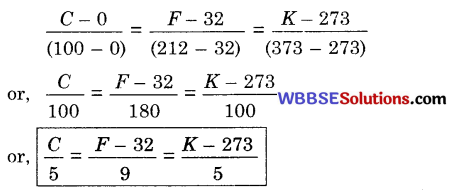Question 6.
Equal masses of milk and water are separately taken in two identical vessels. The vessels are heated simultaneously on the same oven. Which one will have quicker rise of temperature — milk or water and why ?
Explanation: Specific heat of milk is less than that of water, so milk will require a smaller quantity of heat for each degree rise of temperature that needed for the same mass of water. So, supply of heat being same to the equal masses of milk and water, rise of temperature of milk will be quicker than that of water.Numerical problems

Working formula :

$$\frac{C}{5}=\frac{F-32}{9}=\frac{K-273}{5}$$
(Let the temperatures recorded in the Celsius, Fahrenheit and Kelvin thermometers respectively be C, F and K)

(ii) $$1^{\circ} \mathrm{C}=\frac{9^{\circ}}{5} \mathrm{~F} ; 1^{\circ} \mathrm{F}=\frac{5^{\circ}}{9} \mathrm{C}$$

(iii) Relation between temperatures of any two scales, X and Y :
$$\frac{\text { Reading in } X-\text { Lower fixed point of } X}{\text { Fundamental interval of } X}$$
$$=\frac{\text { Reading in } Y-\text { Lower fixed point of } Y}{\text { Fundamental interval of } Y}$$

(iv) Thermal capacity = ms (m = mass of the body ; s = specific heat)

(v) Water equivalent = ms

(vi) Quantity of heat (absorbed or given out) = ms (t2 – t1)
(m – mass ; s = specific heat ;
t1 and t2 are two different temperature)

(vii) Principle of calorimetry :
Heat lost by the hotter body = Heat gained by the colder body

(viii) W = JH (W = work done, H = heat produced, J = mechanical equivalent of heat)Problem 1.
The temperature on a day was 94°F. What is it in celsius scale?
We Know thatProblem 2.
The lowest attainable temperature so far is – 270° celsius. What is it in Fahrenheit scale?
C = -270°
F = ?
We know,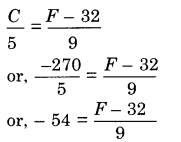or, F – 32 = – 54 x 9 = – 486
or, F= – 486 + 32 – 454
∴ F= – 454°

Problem 3.
Convert 50°C in Fahrenheit scale.
C= 50°
We know,or, F – 32 = 10
or, 90F – 32 × 9
or, F= 90 + 32 = 122
Hence, 50°C = 122°F.Problem 4.
The rise in temperature of a body is 600 in celsius scale. What is the corresponding rise in temperature in Fahrenheit scale?
We know, 1°C = $$\frac{9}{5}$$°F
∴ 60°C = ($$\frac{9}{5}$$ x 60)°F = 108°F

Problem 5.
The difference in the readings of a Fahrenheit and a Celsius thermometer is 480. What are the actual readings shown in the thermometers ?
Let the actual readings in the Fahrenheit and the Celsius thermometers correspond to F and C.
∴ F – C= 48° or, F = C+ 48°
Again, from the relation,or, 9C = 5C + 80 or, 4C 80 or, C = 20
Now, F = C + 48° = 20° + 48° = 68°

Problem 6.
A certain temperature is 104°F, what is its value in Kelvin scale ?
We know the relation between Fahrenheit and Kelvin scale is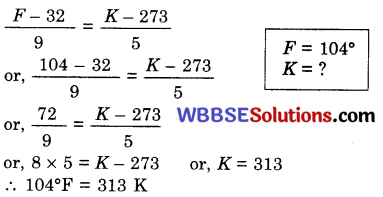Problem 7.
A thermometer having the corresponding upper and lower fixed points 95° and 15°, reads a temperature 65°. What will this temperature be in Celsius scale ?
The relation between temperature of celsius and the given scale can be written from the general relation between any two scales as,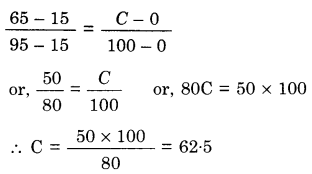∴ The required temperature is 625°C.

Problem 8.
The normal temperature of human body is 98 4°F. What is the value of this temperature in centigrade scale ?
We know,So the required temperature = 3689°C.

Problem 9.
How much calorie of heat would be produced by converting 42 Joule of work completely into heat?
We know, W = JH
or, $$\mathrm{H}=\frac{W}{J}=\frac{42 \mathrm{Joule}}{4 \cdot 2 \mathrm{Joule} / \mathrm{cal}}=10 \mathrm{cal}$$Problem 10.
Which temperature is same in both Centigrade scale and Fahrenheit scale ?
Let the temperature be x°
According to the question, C = F = x
We know,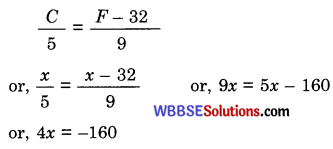or, x = $$\frac{-160}{4}$$ = – 40
So, – 40° temperature will read same both in Fahrenheit and Centigrade scale.

Problem 11.
Mass and specific heat of a substance is 100g and 0.09 respectively. Find the thermal capacity and water equivalent of
the body.
We know,
m = 100g; s = 0.09
Thermal capacity = ms
= 100 × 0.09 cal/°C
Water equivalent = ms
= 100 × 0.09 = 9g

Problem 12.
Calculate the amount of heat required to raise the temperature of 60g of water from 20°C to the boiling point.
m = 50g
t1 = 20°C
t2 = 100°C
s = 1
We know,
Absorbed heat = ms (t2 – t1)
50 × 1 × (100 – 20)
= 50 × 80
= 4000 cal

Problem 13.
A body of iron having mass 60 g is cooled from 200°C to 100°C. Calculate the amount of heat given out. Specific heat of iron = 0.12.
m = 60g
t1 = 100°C
t2 = 200°C
S = 0.12
We know,
the amount of heat given out by iron =
= ms (t2 – t1)
= 60 × 0.12 (200 –  100)
= 60 × 0.12 × 100 = 720 calProblem 14.
300 g lead at 99°C is dropped in 100 g water at 18°C. The final temperature of the mixture is 27°C. Find the specific heat capacity of lead if specific heat capacity of water is 4200 J kg-1 K-1.
Let the specific heat capacity of lead be ‘s’ J/kg/K.
Heat lost by 300 g lead to reach the temperature to 27°C
= $$\frac{300}{1000}$$ = × s × (99 – 27) J
= 0.3 × s × 72 Joules = 21.6s J
Heat gained by 100 g water when the temperature rises from 18°C to 27°C
= 100 × 42000 × (27 – 18) J = 3780 J.
So, from the principle of calorinetry, heat lost heat gained
∴  21.6s = 3780
or, s = $$\frac{3780}{21 \cdot 6}$$ 175 JKg-1 K-1Problem 15.
A piece of copper heated to 35°C is dropped into 140 g of water at 15°C. The mass of copper is 150 g and the specific heat of copper is 400 J/kg/K. Find the final temperature. (Specific heat of water = 4200 J/kg/K)
or, t =$$\frac{60 \times 35+588 \times 15}{648}$$ = 16.85°C